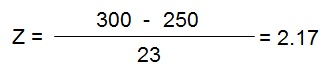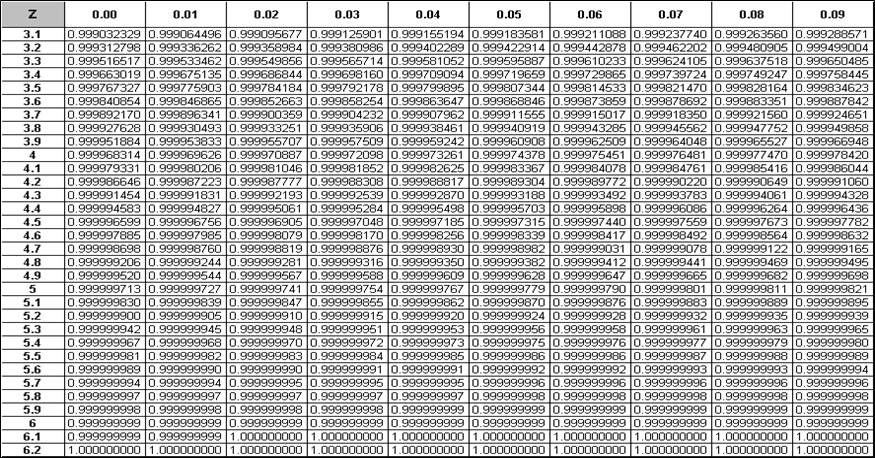# Six Sigma DMAIC Process - Measure Phase - Process Capability

The capability of a process is defined as the inherent variability of a process in the absence of any undesirable special causes and the variability is due to common causes.

Process capability can be categorized under two categories:

Short Term Capability:
Potential performance of a process, under control at a point in time. Calculated from data taken over a short period of time such that there is no external influence on the process (i.e. temperature change, shift change, operator change etc.). Short term capability represents the true process capability. Short term capability indicates the technology of the process.

Long Term Capability:
The actual performance of a process over a period of time. Calculated from data taken over a period of time long enough such that external factors can influence the process. Long term capability represents both the Technological capability combined with the controls that you exercise.

What is Process Sigma?
It is a measurement yardstick to evaluate the output of a process against the set performance standard. Higher the process sigma, better the process capability. Sigma measure gives us a common platform to compare different process that is otherwise being measured differently.

Process Sigma Calculation – Discrete Data:
• What is a Unit – An Item being processed
• What is a Defect – Failure to meet a customer Requirement or a Performance standard
• What is an Opportunity – Any product / service characteristics which is measured to a standard
• What is Defective – A unit that has s defect
• Defects Per Million Opportunity – Number of defects that would arise given a million opportunity
DPMO Calculation:
Defects Per Opportunity
DPO = D / (O*U)
D = Total No of defects
O = Opportunity for defects per unit
U = Total No of Units

DPMO (Defects Per Million Opportunity)
DPMO = 1,000,000 * DPO = 1,000,000 * D/(O*U)

For any Six Sigma process, the calculation will always result the process to have only 3.4 per million opportunities (DPMO). For example, if a process had only 2 Defects, 18 Opportunity for Defects per Unit and Total number of units to be 32500, the DPMO calculation will be as follows:

DPO = 2 / (18*32500) = 0.0000034188
DPMO = DPO * 1000000 = 3.4

Yield:
Different types of fulfillment can impact the quality level we measure in our processes. Yield can be understood as Classical Yield, First Pass Yield and Rolled Throughput Yield.Six Sigma Yield Definition and Example

Classic Yield (YC) – Units Passed / Final Units Tested = 65/70 = 0.93
First Time Yield (Yft) - Units Passed / Units input for First time = 65/100 = 0.65
Rolled Throughput Yield (Ytp) – Yield 1* Yield 2* Yield 3* Yield 4 = (91/100)*(82/91)*(70/82)*(65/70) = 0.65

Process Sigma Calculation – Continuous Data:
Check if the data is Normally distributed. The Larger the sample size, the higher the probability of having normal data. In normality plot the Y axis represents the cumulative percentage if the data points that fall below the corresponding data value on the X axis.Six Sigma Normal Probability Plot

Calculation of Z value:
Z is the unit of measure that is equivalent to the number of Standard Deviation a value is away from the mean value.Calculation of Six Sigma Z Value

Y = Value of the data point we are concerned with
µ = Mean of the data points
σ = Standard Deviation of the data points
Z = Number of standard deviations between Y & the mean (µ)

Let’s look at some examples:
It’s found that runs scored by England cricket team while setting a score in one day internationals follow a normal distribution with mean of 250 & standard deviation of 23. What is the probability that team will score more than 300 runs in its next match?Normal Distribution ExampleZ Calculation Example

Looking up Appendix below for Normal Distribution Table, we find that Z value of 2.17 covers an area of 0.98499 under itself. Thus, the probability that the team may score between 0 & 300 is 98.5% & thus, chance of team scoring more than 300 runs is 1.5%.

For the same data, what is the probability that team will score between 216 & 273?Normal Distribution ExampleZ Calculation Example

From Appendix:
Total area covered by Z1 = 0.841344740
Total area covered by Z2 = 1 - 0.929219087 = 0.0707
Intercepted area between Z1 & Z2 = 0.7705
Thus, probability that the team may score between 216 & 273 runs in the next match is 77.05%.

Appendix - Normal Distribution TablesSix Sigma Normal Distribution Table 1Six Sigma Normal Distribution Table 2

# We guarantee that Your Free Online Training will make you pass Your Six Sigma Certification Exam!